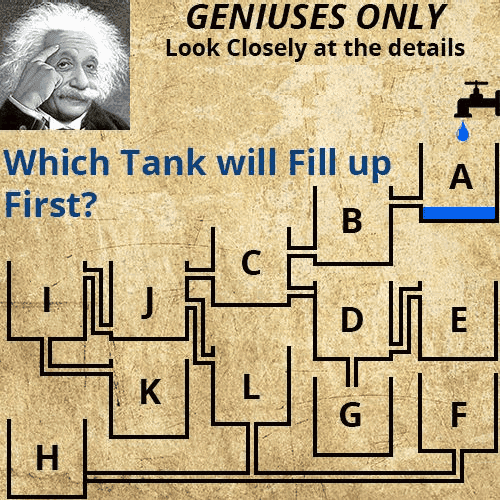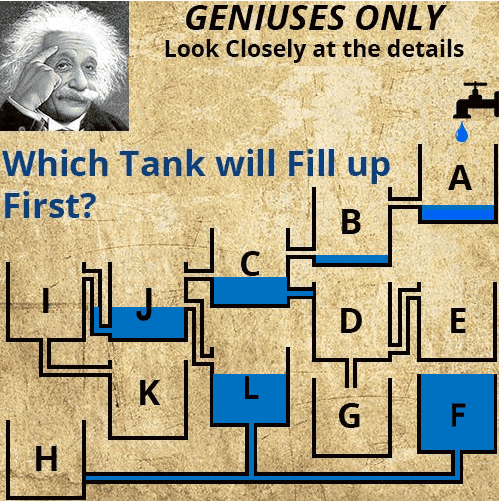# Which tank fills first

• II originally thought tank K because the area in tank J is much larger than the area in the pipe connecting J to I
But then I realize Bernoulli's equation does not include volume but only includes height.
H_1, H_2 = height of water tank J, height of water in pipe between tanks J and I
P, p, V = pressure, density, velocity
$$P_1+pgH_1+\frac{1}{2}pV_1^2=P_2+pgH_2+\frac{1}{2}pV_2^2$$
Pressure terms are the same, so is density. Velocity = 0, gravity cancels out ##H_1=H_2##
So even in the small pipe connecting J to I, the water level is going to be the same as the height of water in the tank J.

Is this correct?

Also note the pipe from C to D is blocked off.
Also I assume A would be trivial solution so water must exit pipe faster than the tap fills tank A

## Answers and Replies

jbriggs444
Science Advisor
Homework Helper
The water is dripping in. Flow velocity will be negligible.

•FactChecker
Regla
It really depends on what point do you consider the tank is 'full', let's say that the point full is when the tank is overfilling. I think the tank H would get filled first because the connection JI goes higher than connection JL.

I say F will fill up (overflowing) first!

•Jon Richfield, davenn, Merlin3189 and 4 others
jbriggs444
Science Advisor
Homework Helper
It really depends on what point do you consider the tank is 'full', let's say that the point full is when the tank is overfilling. I think the tank H would get filled first because the connection JI goes higher than connection JL.
There is no path into tank H.

Regla
There is no path into tank H.
whoops that's true. Then my guess is F. Thanks.

•FactChecker, jonk75 and sophiecentaur
CWatters
Science Advisor
Homework Helper
Gold Member
Tank G fills up first. The water flows A B C D G. No water goes into J. No water goes into E.

Darn I didn't spot the blocked pipe. Ok so yes it's F.

•shihab-kol, jonk75, sophiecentaur and 1 other person
phinds
Science Advisor
Gold Member
It's G

•RandyD123
Nugatory
Mentor
It's G
You've missed the blocked pipe C to D.

Nugatory
Mentor
A and B fill to the level of their outputs; C fills to the level of its outlet into J; J fills to the level of its outlet into L; L fills to the level of the top of F; and F fills and overflows. Once F is full, every drop of water into A causes another drop to overflow from F and the system is in steady state.

•sophiecentaur and russ_watters
phinds
Science Advisor
Gold Member
You've missed the blocked pipe C to D.
Damn ! Thanks.

CWaters even SAID it was there and I still couldn't see it.A and B fill to the level of their outputs; C fills to the level of its outlet into J; J fills to the level of its outlet into L; L fills to the level of the top of F; and F fills and overflows. Once F is full, every drop of water into A causes another drop to overflow from F and the system is in steady state.

So, basically, the area doesn't matter of tank J, it wont push the water through the pipe higher than its own water level? Tank J is roughly 27 times the cross sectional area then the pipe... but it doesn't matter right?

Nugatory
Mentor
So, basically, the area doesn't matter of tank J, it wont push the water through the pipe higher than its own water level? Tank J is roughly 27 times the cross sectional area then the pipe... but it doesn't matter right?
Not as long as the rate at which water enters the tank is small compared with the rate at which a single pipe can carry the water out - the water level will not rise above the height of the lowest flowing pipe. The illustration suggests that we're dealing with a dripping faucet which will easily be handled by a single pipe - think about an air conditioning unit's condensate drain tray.

•fahraynk
It's K

Nugatory
Mentor
It's K
Can't be - nothing flows from J to I because the level in J doesn't rise above the outlet from J to L.

•davenn
Stressil
I choose L due to pressure and flow

RonL
Gold Member
I say F will fill up (overflowing) first!
I agree with you, F will overflow before any others•davenn
davenn
Science Advisor
Gold Member
I choose L due to pressure and flow

no ... look again .... F is at a lower level than L which fills F

Like phinds and a couple of others, I originally was going for G as I didn't see the block between C and D

DrGreg
Science Advisor
Gold Member
Here's the picture for the slow-drip steady-state solution:•Rajini, DrClaude, RandyD123 and 5 others
View attachment 208203

I originally thought tank K because the area in tank J is much larger than the area in the pipe connecting J to I
But then I realize Bernoulli's equation does not include volume but only includes height.
H_1, H_2 = height of water tank J, height of water in pipe between tanks J and I
P, p, V = pressure, density, velocity
$$P_1+pgH_1+\frac{1}{2}pV_1^2=P_2+pgH_2+\frac{1}{2}pV_2^2$$
Pressure terms are the same, so is density. Velocity = 0, gravity cancels out ##H_1=H_2##
So even in the small pipe connecting J to I, the water level is going to be the same as the height of water in the tank J.

Is this correct?

Also note the pipe from C to D is blocked off.
Also I assume A would be trivial solution so water must exit pipe faster than the tap fills tank A

Tank F will fill up first

The Wizard
Flow Chart is A - B - C - J - L - F

No tank will fill before F and as F is open at the top it will constantly overflow, therefore no other Tank can fill fully.

F fills first; L prevents any flow reaching I from J because inlet to I is higher than outlet to L. J must fill before any tank above (A, B, or C).
BUT ASSUMPTIONS ARE EVERYTHING.
F fills first only if 1) all the pipes are the same diameter and of the same material, 2) the heights of all tanks are identical, 3) the flow from the faucet does not exceed the pipe capacity (as indicated by the drip), and 4) therefore, flow through system is not sufficient to impact dynamic pressure caused by line friction and elbows. With these assumptions, the only law needed is water seeking lowest level.

Once you violate any of these assumptions, you will need to work through the system performance using the Bernoulli's equation; and the complexity of the system increases substantially, probably requiring a full dynamic modeling exercise.

EXAMPLE: Let's just change one assumption: that the faucet inflow exceeds the outflow capacity of Tank A, even when tank A is full.
When the faucet flow rate exceeds the outflow pipe capacity, A will begin to fill to over flowing. As it does, the pressure at the outlet will increase, thereby increasing the outflow rate. Thus, the inflow to i B will be greater than the outflow from B to C, and B will begin to fill. Since the outlet from B is lower relative to the tank height than is the outlet from tank A, at some point, the outflow from B may match that from A. The height difference of the two tanks as well as the depth of the outlet of the tank will determine whether B will over flow. It does appear that tank B is taller than tank A and that the A outlet is not as deep at the B outlet, thus the level in B will stabilize below over flow. This same exercise must now proceed through each box.

Reference https://www.physicsforums.com/threads/which-tank-fills-first.921715/

No liquid will ever reach D, E or G....due to the closed off pipe from C to D.....so none of them

View attachment 208203

I originally thought tank K because the area in tank J is much larger than the area in the pipe connecting J to I
But then I realize Bernoulli's equation does not include volume but only includes height.
H_1, H_2 = height of water tank J, height of water in pipe between tanks J and I
P, p, V = pressure, density, velocity
$$P_1+pgH_1+\frac{1}{2}pV_1^2=P_2+pgH_2+\frac{1}{2}pV_2^2$$
Pressure terms are the same, so is density. Velocity = 0, gravity cancels out ##H_1=H_2##
So even in the small pipe connecting J to I, the water level is going to be the same as the height of water in the tank J.

Is this correct?

Also note the pipe from C to D is blocked off.
Also I assume A would be trivial solution so water must exit pipe faster than the tap fills tank A
Through observation, looks like G would fill first' others will just move the water to other places
IMHO

jbriggs444
Science Advisor
Homework Helper
Through observation, looks like G would fill first' others will just move the water to other places
IMHO
If you review the original post in this thread you will see why that is not correct. Several others have already missed seeing:
Also note the pipe from C to D is blocked off.

•fahraynk